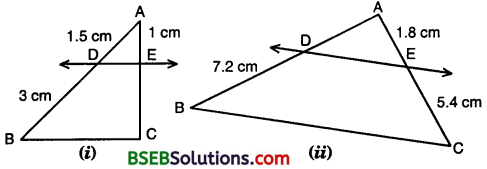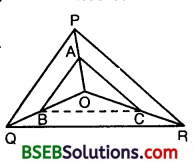# Bihar Board Class 10th Maths Solutions Chapter 6 Triangles Ex 6.2

Bihar Board Class 10th Maths Solutions Chapter 6 Triangles Ex 6.2 Textbook Questions and Answers.

## BSEB Bihar Board Class 10th Maths Solutions Chapter 6 Triangles Ex 6.2Question 1.
In figures, (i) and (ii), DE || BC. Find EC in (i) and AD in (ii).Solution:
(i) In Fig. (i),
since DE || BC, therefore
$$\frac { AD }{ DB }$$ = $$\frac { AE }{ EC }$$ or $$\frac { 1.5 }{ 3 }$$ = $$\frac { 1}{ EC }$$
or EC = $$\frac { 3}{ 1.5}$$ = $$\frac{3 \times 10}{15}$$ Cm = 2 cm

(ii) In Fig. (ii),
since DE || BC, therefore
$$\frac { AD }{ DB }$$ =$$\frac { AE }{ EC}$$ or $$\frac { AD }{7.2 }$$ = $$\frac { 1.8 }{ 5.4 }$$
or AD = $$\frac { 18 }{ 54 }$$ x $$\frac { 72 }{ 10 }$$ cm = 2.4 cm.

Question 2.
E and F are points on the sides PQ and PR respectively of a A PQR. For each of the following cases, state whether EF || QR :
(i) PE = 3.9 cm, EQ = 3 cm, PF = 3.6 cm and FR = 2.4 cm
(ii) PE = 4 cm, QE = 4.5 cm, PF = 8 cm and RF = 9 cm
(iii) PQ = 1.28 cm, PR = 2.56 cm, PE = 0.18 cm and PF = 0.36 cm
Solution:
(i) We have :
PE = 3.9 cm, EQ = 3 cm,
PF = 3.6 cm and FR = 2.4 cmi. e., EF does not divide the sides PQ and PR of A PQR in the same ratio. Therefore, EF is not parallel to QR.

(ii) We have : PE = 4 cm, QE = 4.5 cm, PF = 8 cm and RF = 9 cm
Now, $$\frac { PE }{ EQ }$$ = $$\frac { 4 }{ 4.5 }$$ = $$\frac { 40 }{ 45 }$$ = $$\frac { 8 }{ 9 }$$
and $$\frac { PF }{ FR }$$ = $$\frac { 8 }{ 9 }$$
So, $$\frac { PE }{ EQ }$$ = $$\frac { PF }{ FR }$$
Thus, EF divides sides PQ and PR of ∆ PQR in the same ratio. Therefore, by the converse of Basic Proportionality Theorem, we have EF || QR.

(iii) We have :PQ= 1.28 cm, PR = 2.56 cm,
PE = 0.18 cm and PF 0.36 cm
∴ EQ = PQ – PE = (1.28 – 0.18) cm = 1.10 cm
and FR = PR – PF = (2.56 – 0.36) = 2.20 cm
Now, $$\frac { PE }{ EQ }$$ = $$\frac { 0.18 }{ 1.10 }$$ = $$\frac { 18 }{ 110 }$$ = $$\frac { 9 }{ 55 }$$
and $$\frac { PF }{ FR }$$ = $$\frac { 0.36 }{ 2.20 }$$ = $$\frac { 36 }{ 220 }$$ = $$\frac { 9 }{ 55 }$$
So, $$\frac { PE }{ EQ }$$ = $$\frac { PF }{ FR }$$
Thus, EF divides sides PQ and PR of ∆ PQR in the same ratio. Therefore, by the converse of Basic Proportionality Theorem, we have EF || QR.Question 3.
In the figure, if LM || CB and LN || CD, prove that $$\frac { AM }{ AB }$$ = $$\frac { AN }{ AD }$$Solution:
In ∆ ABC, we have :
LM || CB
∴By a result based on Basic Proportionality Theorem (a corollary), we have :
$$\frac { AM }{ AB }$$ = $$\frac { AL }{ AC }$$ … (1)
In ∆ ACD, we have :
LN || CD [Given]
∴By a result based on Basic Proportionality Theorem, we have :
$$\frac { AL }{ AC }$$ = $$\frac { AN }{ AD }$$
From (1) and (2), we obtain that
$$\frac { AM }{ AB }$$ = $$\frac { AN }{ AD }$$

Question 4.
In the figure, DE || AC and DF || AE. Prove that $$\frac { BF }{ FE }$$ = $$\frac { BE }{ EC }$$Solution:
In ∆ BGA, we have :
DE || ‘AC
∴By Basic Proportionality Theorem, we have :
$$\frac { BE }{ EC }$$ = $$\frac { BD }{ DA }$$ …. (1)
In ∆ BEA, we have :
DF || AE [Given]
∴By Basic Proportionality Theorem, we have
$$\frac { BF }{ FE }$$ = $$\frac { BD }{ DA }$$ …. (2)
From (1) and (2), we obtain that
$$\frac { BF }{ FE }$$ = $$\frac { BE }{ EC }$$.

Question 5.
In the figure, DE || OQ and DF || OR. Show that EF || QR.Solution:
In ∆ PQO, we have :
DE || OQ [Given]
∴By Basic Proportionality Theorem, we have :
$$\frac { PE }{ EQ }$$ = $$\frac { PD }{ DO }$$ … (1)
In ∆ POR, We have :
DF || OR [Given]
∴By Basic Proportionality Theorem, we have
$$\frac { PD }{ DO }$$ = $$\frac { PF }{ FR }$$ … (2)
From (1) and (2), we obtain that
$$\frac { PE }{ EQ }$$ = $$\frac { PF }{ FR }$$
So, EF || QR [By the converse of BPT]Question 6.
In the figure, A, B and C are points on OP, OQ and OR respectively such that AB || PQ and AC || PR. Show that BC || QR.Solution:
Given : O is any point within ∆ PQR, AB || PQ and AC || PR
To prove : BC || QR.
Construction : Join BC.
Proof:
In ∆ OPQ, we have :
AB || PQ [Given]
∴By Basic Proportionality Theorem, we have :
$$\frac { OA }{ AP }$$ = $$\frac { OB }{ BQ }$$ … (1)
In ∆ OPR, We have :
AC || PR [Given]
∴ By Basic Proportionality Theorem, we have
$$\frac { OA }{ AP }$$ = $$\frac { OC }{ CR }$$ … (2)
From (1) and (2), we obtain that
$$\frac { OB }{ BQ }$$ = $$\frac { OC }{ CR }$$
Thus, in ∆ OQR, B and C are points dividing the sides OQ and OR in the same ratio. Therefore, by the converse of Basic Proportionality Theorem, we have :
BC || QR.

Question 7.
Using Theorem 6.1, prove that a line drawn through the mid-point of one side of a triangle parallel to another side bisects the third side. (Recall that you have proved it in Class IX).Solution:
Given : ∆ ABC, in which D is the mid-point of side AB and the line DE is drawn parallel to BC, meeting AC in E.
To prove : AE = EC
Proof:
Since DE || BC, therefore by Basic Proportionality Theorem, we have :
$$\frac { AD }{ DB }$$ = $$\frac { AE }{ EC }$$ … (1)
But AD = DB [∵D is the mid-point of AB]
i.e., $$\frac { AD }{ DB }$$ = 1
∴ From (1), $$\frac { AE }{ EC }$$ = 1 or AE = EC
Hence, E is the mid-point of the third side AC.Question 8.
Using Theorem 6.2, prove that the line joining the mid-points of any two sides of a triangle is parallel to the third side. (Recall that you have done it in Class IX).Solution:
Given : ∆ ABC, in which D and E are the mid¬points of sides AB and AC respectively.
To prove : DE || BC.
Proof :
Since D and E are the mid-points of AB and AC respectively, therefore
AD = DB and AE = EC
∴ $$\frac { AD }{ DB }$$ = 1
and AE = EC
∴ $$\frac { AE }{ EC }$$ = 1
Thus, in ∆ ABC, D and E are points dividing the sides AB and AC in the same ratio. Therefore, by the converse of Basic Proportionality Theorem (Theorem 6.2), we have :
DE || BC.

Question 9.
ABCD is a trapezium in which AB || DC and its diagonals intersect each other at the point O. Show that $$\frac { AO }{ BO }$$ = $$\frac { CO }{ DO }$$.
Solution:
Given : A trapezium ABCD, in which AB || DC and its diagonals AC and BD intersect each other at O.
To prove : $$\frac { AO }{ BO }$$ = $$\frac { CO }{ DO }$$.
Construction : Through O, draw OE || AB, i.e., OE || DC.
Proof:
In ∆ ADC, we have :
OE || DC [Construction]
∴ By Basic Proportionality Theorem, we have :$$\frac { AE }{ ED }$$ = $$\frac { AO }{ CO }$$ … (1)
Again, in ∆ ABD, we have :
OE || AB [Construction]
∴By Basic Proportionality Theorem, we have :0
$$\frac { ED }{ AE }$$ = $$\frac { DO }{ BO }$$ or $$\frac { AE }{ ED }$$ = $$\frac { BO }{ DO }$$ … (2)
From (1) and (2), we obtain that
$$\frac { AO }{ CO }$$ = $$\frac { BO }{ DO }$$ or $$\frac { AO }{ BO }$$ = $$\frac { CO }{ DO }$$.Question 10.
The diagonals of a quadrilateral ABCD intersect each other at the point O such that $$\frac { AO }{ BO }$$ = $$\frac { CO }{ DO }$$ that ABCD is a trapezium.
Solution:
Given : A quadrilateral ABCD in which its diagonals AC and BD intersect each other at the point O such that $$\frac { AO }{ BO }$$ = $$\frac { CO }{ DO }$$, i.e., $$\frac { AO }{ CO }$$ = $$\frac { BO }{ DO }$$
To prove : Quadrilateral ABCD is a trapezium.
Construction : Through O, draw OE || AB meeting AD in E.
Proof:
In ∆ ADB, we have :
OE || AB [Construction]
∴ By Basic Proportionality Theorem, we have :
$$\frac { DE }{ EA }$$ = $$\frac { OD }{ BO }$$
or $$\frac { EA }{ DE }$$ = $$\frac { BO }{ DO }$$
i.e., $$\frac { EA }{ DE }$$ = $$\frac { BO }{ DO }$$ = $$\frac { AO }{ CO }$$ [ ∵$$\frac { AO }{ CO }$$ = $$\frac { BO }{ DO }$$(given)]
or $$\frac { EA }{ DE }$$ = $$\frac { AO }{ CO }$$
Thus, in ∆ ADC, points E and O are dividing the sides AD and AC in the same ratio. Therefore, by the converse of Basic Proportionality Theorem, we have :
EO || DC
But, EO || AB [construction]
Hence, AB || DC
∴ Quadrilateral ABCD is a trapezium